AP State Board Syllabus AP SSC 10th Class Maths Textbook Solutions Chapter 10 Mensuration Ex 10.1 Textbook Questions and Answers.

## AP State Syllabus SSC 10th Class Maths Solutions 10th Lesson Mensuration Exercise 10.1

### 10th Class Maths 10th Lesson Mensuration Ex 10.1 Textbook Questions and Answers

Question 1.
A joker’s cap is in the form of right circular cone whose base radius is 7 cm and height is 24 cm. Find the area of the sheet required to make 10 such caps.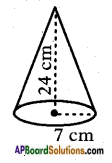Radius of the cap (r) = 7 cm
Height of the cap (h) = 24 cm
Slant height of the cap (l) = $$\sqrt{r^{2}+h^{2}}$$
= $$\sqrt{7^{2}+24^{2}}$$
= $$\sqrt{49+576}$$
= √625
= 25
∴ l = 25 cm.
Lateral surface area of the cap = Cone = πrl
L.S.A. = $$\frac{22}{7}$$ × 7 × 25 = 550 cm2.
∴ Area of the sheet required for 10 caps = 10 x 550 = 5500 cm2.Question 2.
A sports company was ordered to prepare 100 paper cylinders without caps for shuttle cocks. The required dimensions of the cylinder are 35 cm length / height and its radius is 7 cm. Find the required area of thin paper sheet needed to make 100 cylinders.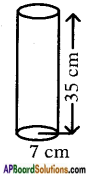Radius of the cylinder, r = 7 cm
Height of the cylinder, h = 35 cm
T.S.A. of the cylinder with lids at both ends = 2πr(r+h)
= 2 × $$\frac{22}{7}$$ × 7 × (7 + 35)
= 2 × $$\frac{22}{7}$$ × 7 × 42 = 1848 cm2.
Area of thin paper required for 100 cylinders = 100 × 1848
= 184800 cm2
= $$\frac{184800}{100 \times 100}$$ m2
= 18.48 m2.

Question 3.
Find the volume of right circular cone with radius 6 cm. and height 7 cm.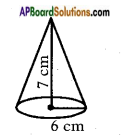Base radius of the cone (r) = 6 cm.
Height of the cone (h) = 7 cm
Volume of the cone = $$\frac{1}{3}$$πr2h
= $$\frac{1}{3}$$ × $$\frac{22}{7}$$ × 6 × 6 × 7
= 264 c.c. (Cubic centimeters)
∴ Volume of the right circular cone = 264 c.c.Question 4.
The lateral surface area of a cylinder is equal to the curved surface area of a cone. If their base be the same, find the ratio of the height of the cylinder to slant height of the cone.
Base of cylinder and cone be the same.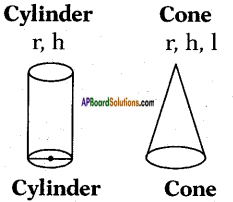CSA / LSA of cylinder = 2πrh
CSA of cone = πrl
The lateral surface area of a cylinder is equal to the curved surface area of cone.
∴ 2πrh = πrl
⇒ $$\frac{h}{l}=\frac{\pi r}{2 \pi r}$$
⇒ $$\frac{h}{l}$$ = $$\frac{1}{2}$$
∴ h : l = 1 : 2

Question 5.
A self help group wants to manufacture joker’s caps (conical caps) of 3 cm radius and 4 cm height. If the available colour paper sheet is 1000 cm2, then how many caps can be manufactured from that paper sheet?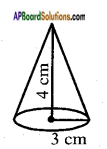Radius of the cap (conical cap) (r) = 3 cm
Height of the cap (h) = 4 cm
Slant height l = $$\sqrt{r^{2}+h^{2}}$$
(by Pythagoras theorem)
= $$\sqrt{3^{2}+4^{2}}$$
= $$\sqrt{9+16}$$
= √25
= 5 cm
C.S.A. of the cap = πrl
= $$\frac{22}{7}$$ × 3 × 5
≃ 47.14 cm2
Number of caps that can be made out of 1000 cm2 = $$\frac{1000}{47.14}$$ ≃ 21.27
∴ Number of caps = 21.Question 6.
A cylinder and cone have bases of equal radii and are of equal heights. Show that their volumes are in the ratio of 3 : 1.
Given dimensions are:
Cone:
Height = h
Volume (V) = $$\frac{1}{3}$$πr2h

Cylinder:
Height = h
Volume (V) = πr2h

Ratio of volumes of cylinder and cone = πr2h : $$\frac{1}{3}$$πr2h
= 1 : $$\frac{1}{3}$$
= 3 : 1
Hence, their volumes are in the ratio = 3 : 1.

Question 7.
A solid iron rod has cylindrical shape. Its height is 11 cm. and base diameter is 7 cm. Then find the total volume of 50 rods?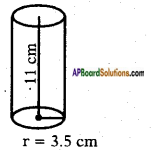Diameter of the cylinder (d) = 7 cm
Radius of the base (r) = $$\frac{7}{2}$$ = 3.5 cm
Height of the cylinder (h) = 11 cm
Volume of the cylinder V = πr2h
= $$\frac{22}{7}$$ × 3.5 × 3.5 × 11 = 423.5 cm3
∴ Total volume of 50 rods = 50 × 423.5 cm3 = 21175 cm3.Question 8.
A heap of rice is in the form of a cone of diameter 12 m. and height 8 m. Find its volume? How much canvas cloth is required to cover the heap? (Use π = 3.14)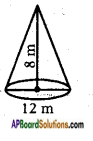Diameter of the heap (conical) (d) = 12 cm
∴ Radius = $$\frac{d}{2}$$ = $$\frac{12}{2}$$ = 6 cm
Height of the cone (h) = 8 m
Volume of the cone, V = $$\frac{1}{3}$$πr2h
= $$\frac{1}{3}$$ × $$\frac{22}{7}$$ × 6 × 6 × 8
= 301.71 m3.

Question 9.
The curved surface area of a cone is 4070 cm2 and its diameter is 70 cm. What is its slant height?
Radius of the cone = r = $$\frac{d}{2}$$ = $$\frac{70}{2}$$ = 35 cm
$$\frac{22}{7}$$ × 35 × l = 4070
l = $$\frac{4070}{110}$$ = 37 cm